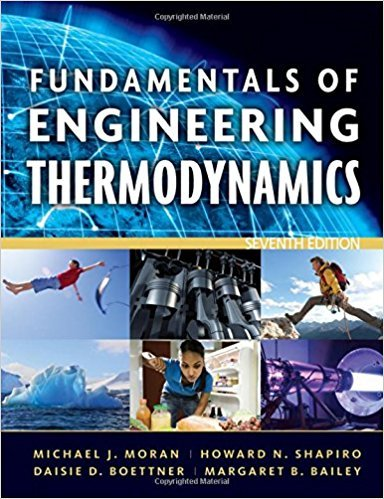×
Get Full Access to Fundamentals Of Engineering Thermodynamics - 7 Edition - Chapter 2 - Problem 3p
Get Full Access to Fundamentals Of Engineering Thermodynamics - 7 Edition - Chapter 2 - Problem 3p

×

# Exploring Energy ConceptsAn object whose weight is 100 lbfISBN: 9780470495902 50

## Solution for problem 3P Chapter 2

Fundamentals of Engineering Thermodynamics | 7th Edition

• Textbook Solutions
• 2901 Step-by-step solutions solved by professors and subject experts
• Get 24/7 help from StudySoup virtual teaching assistantsFundamentals of Engineering Thermodynamics | 7th Edition

4 5 1 359 Reviews
31
5
Problem 3P

Problem 3P

Exploring Energy Concepts

An object whose weight is 100 lbf experiences a decrease in kinetic energy of 500 ft · lbf and an increase in potential energy of 1500 ft · lbf. The initial velocity and elevation of the object, each relative to the surface of the earth, are 40 ft/s and 30 ft, respectively. If g = 32.2 ft/s2, determine

(a) the final velocity, in ft/s.

(b) the final elevation, in ft.

Step-by-Step Solution:

Solution

Step 1 of 3

Consider an object whose weight iswhich experience a decrease in kinetic energyand an increase in potential energy of.

We need to find out the final velocity and the final elevation inif the initial velocity relative to the surface of the earth of the object isand the initial elevation relative to the surface of the earth of the object is.

Step 2 of 3

Step 3 of 3

##### ISBN: 9780470495902

Unlock Textbook Solution